# predict

Class: ClassificationLinear

Predict labels for linear classification models

## Syntax

``Label = predict(Mdl,X)``
``Label = predict(Mdl,X,'ObservationsIn',dimension)``
``````[Label,Score] = predict(___)``````

## Description

example

````Label = predict(Mdl,X)` returns predicted class labels for each observation in the predictor data `X` based on the trained, binary, linear classification model `Mdl`. `Label` contains class labels for each regularization strength in `Mdl`.```

example

````Label = predict(Mdl,X,'ObservationsIn',dimension)` specifies the predictor data observation dimension, either `'rows'` (default) or `'columns'`. For example, specify `'ObservationsIn','columns'` to indicate that columns in the predictor data correspond to observations.```

example

``````[Label,Score] = predict(___)``` also returns classification scores for both classes using any of the input argument combinations in the previous syntaxes. `Score` contains classification scores for each regularization strength in `Mdl`.```

## Input Arguments

expand all

Binary, linear classification model, specified as a `ClassificationLinear` model object. You can create a `ClassificationLinear` model object using `fitclinear`.

Predictor data to be classified, specified as a full or sparse numeric matrix or a table.

By default, each row of `X` corresponds to one observation, and each column corresponds to one variable.

• For a numeric matrix:

• The variables in the columns of `X` must have the same order as the predictor variables that trained `Mdl`.

• If you train `Mdl` using a table (for example, `Tbl`) and `Tbl` contains only numeric predictor variables, then `X` can be a numeric matrix. To treat numeric predictors in `Tbl` as categorical during training, identify categorical predictors by using the `CategoricalPredictors` name-value pair argument of `fitclinear`. If `Tbl` contains heterogeneous predictor variables (for example, numeric and categorical data types) and `X` is a numeric matrix, then `predict` throws an error.

• For a table:

• `predict` does not support multicolumn variables or cell arrays other than cell arrays of character vectors.

• If you train `Mdl` using a table (for example, `Tbl`), then all predictor variables in `X` must have the same variable names and data types as the variables that trained `Mdl` (stored in `Mdl.PredictorNames`). However, the column order of `X` does not need to correspond to the column order of `Tbl`. Also, `Tbl` and `X` can contain additional variables (response variables, observation weights, and so on), but `predict` ignores them.

• If you train `Mdl` using a numeric matrix, then the predictor names in `Mdl.PredictorNames` must be the same as the corresponding predictor variable names in `X`. To specify predictor names during training, use the `PredictorNames` name-value pair argument of `fitclinear`. All predictor variables in `X` must be numeric vectors. `X` can contain additional variables (response variables, observation weights, and so on), but `predict` ignores them.

Note

If you orient your predictor matrix so that observations correspond to columns and specify `'ObservationsIn','columns'`, then you might experience a significant reduction in optimization execution time. You cannot specify `'ObservationsIn','columns'` for predictor data in a table.

Data Types: `table` | `double` | `single`

Predictor data observation dimension, specified as `'columns'` or `'rows'`.

Note

If you orient your predictor matrix so that observations correspond to columns and specify `'ObservationsIn','columns'`, then you might experience a significant reduction in optimization execution time. You cannot specify `'ObservationsIn','columns'` for predictor data in a table.

## Output Arguments

expand all

Predicted class labels, returned as a categorical or character array, logical or numeric matrix, or cell array of character vectors.

The `predict` function classifies an observation into the class yielding the highest score. For an observation with `NaN` scores, the function classifies the observation into the majority class, which makes up the largest proportion of the training labels.

In most cases, `Label` is an n-by-L array of the same data type as the observed class labels (`Y`) used to train `Mdl`. (The software treats string arrays as cell arrays of character vectors.) n is the number of observations in `X` and L is the number of regularization strengths in `Mdl.Lambda`. That is, `Label(i,j)` is the predicted class label for observation `i` using the linear classification model that has regularization strength `Mdl.Lambda(j)`.

If `Y` is a character array and L > 1, then `Label` is a cell array of class labels.

Classification scores, returned as a n-by-2-by-L numeric array. n is the number of observations in `X` and L is the number of regularization strengths in `Mdl.Lambda`. `Score(i,k,j)` is the score for classifying observation `i` into class `k` using the linear classification model that has regularization strength `Mdl.Lambda(j)`. `Mdl.ClassNames` stores the order of the classes.

If `Mdl.Learner` is `'logistic'`, then classification scores are posterior probabilities.

## Examples

expand all

`load nlpdata`

`X` is a sparse matrix of predictor data, and `Y` is a categorical vector of class labels. There are more than two classes in the data.

The models should identify whether the word counts in a web page are from the Statistics and Machine Learning Toolbox™ documentation. So, identify the labels that correspond to the Statistics and Machine Learning Toolbox™ documentation web pages.

`Ystats = Y == 'stats';`

Train a binary, linear classification model using the entire data set, which can identify whether the word counts in a documentation web page are from the Statistics and Machine Learning Toolbox™ documentation.

```rng(1); % For reproducibility Mdl = fitclinear(X,Ystats);```

`Mdl` is a `ClassificationLinear` model.

Predict the training-sample, or resubstitution, labels.

`label = predict(Mdl,X);`

Because there is one regularization strength in `Mdl`, `label` is column vectors with lengths equal to the number of observations.

Construct a confusion matrix.

`ConfusionTrain = confusionchart(Ystats,label);`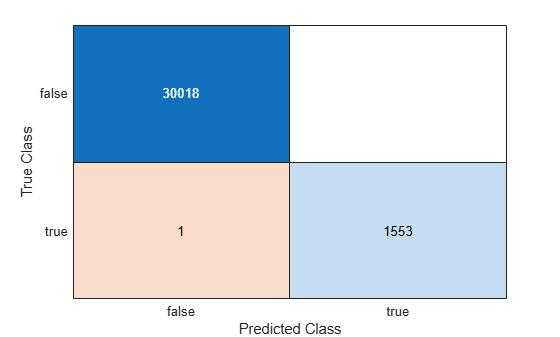The model misclassifies only one `'stats'` documentation page as being outside of the Statistics and Machine Learning Toolbox documentation.

Load the NLP data set and preprocess it as in Predict Training-Sample Labels. Transpose the predictor data matrix.

```load nlpdata Ystats = Y == 'stats'; X = X';```

Train a binary, linear classification model that can identify whether the word counts in a documentation web page are from the Statistics and Machine Learning Toolbox™ documentation. Specify to hold out 30% of the observations. Optimize the objective function using SpaRSA.

```rng(1) % For reproducibility CVMdl = fitclinear(X,Ystats,'Solver','sparsa','Holdout',0.30,... 'ObservationsIn','columns'); Mdl = CVMdl.Trained{1};```

`CVMdl` is a `ClassificationPartitionedLinear` model. It contains the property `Trained`, which is a 1-by-1 cell array holding a `ClassificationLinear` model that the software trained using the training set.

Extract the training and test data from the partition definition.

```trainIdx = training(CVMdl.Partition); testIdx = test(CVMdl.Partition);```

Predict the training- and test-sample labels.

```labelTrain = predict(Mdl,X(:,trainIdx),'ObservationsIn','columns'); labelTest = predict(Mdl,X(:,testIdx),'ObservationsIn','columns');```

Because there is one regularization strength in `Mdl`, `labelTrain` and `labelTest` are column vectors with lengths equal to the number of training and test observations, respectively.

Construct a confusion matrix for the training data.

`ConfusionTrain = confusionchart(Ystats(trainIdx),labelTrain);`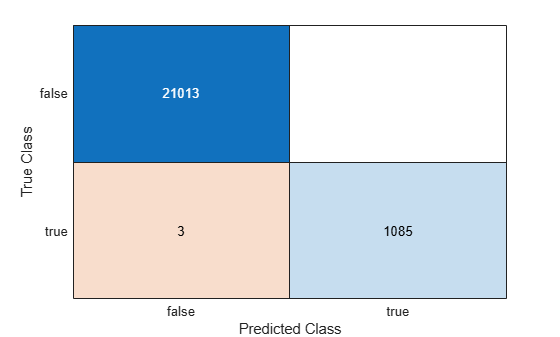The model misclassifies only three documentation pages as being outside of Statistics and Machine Learning Toolbox documentation.

Construct a confusion matrix for the test data.

`ConfusionTest = confusionchart(Ystats(testIdx),labelTest);`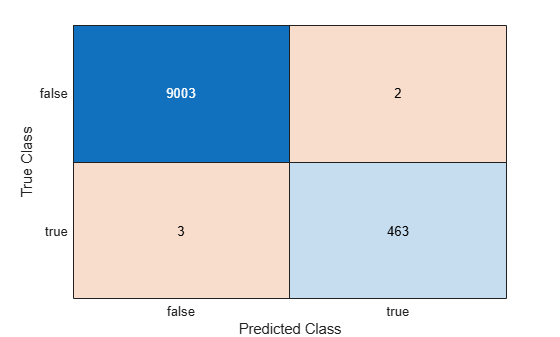The model misclassifies three documentation pages as being outside the Statistics and Machine Learning Toolbox, and two pages as being inside.

Estimate test-sample, posterior class probabilities, and determine the quality of the model by plotting a receiver operating characteristic (ROC) curve. Linear classification models return posterior probabilities for logistic regression learners only.

Load the NLP data set and preprocess it as in Predict Test-Sample Labels.

```load nlpdata Ystats = Y == 'stats'; X = X';```

Randomly partition the data into training and test sets by specifying a 30% holdout sample. Identify the test-set indices.

```cvp = cvpartition(Ystats,'Holdout',0.30); idxTest = test(cvp);```

Train a binary linear classification model. Fit logistic regression learners using SpaRSA. To hold out the test set, specify the partitioned model.

```CVMdl = fitclinear(X,Ystats,'ObservationsIn','columns','CVPartition',cvp,... 'Learner','logistic','Solver','sparsa'); Mdl = CVMdl.Trained{1};```

`Mdl` is a `ClassificationLinear` model trained using the training set specified in the partition `cvp` only.

Predict the test-sample posterior class probabilities.

`[~,posterior] = predict(Mdl,X(:,idxTest),'ObservationsIn','columns');`

Because there is one regularization strength in `Mdl`, `posterior` is a matrix with 2 columns and rows equal to the number of test-set observations. Column i contains posterior probabilities of `Mdl.ClassNames(i)` given a particular observation.

Compute the performance metrics (true positive rates and false positive rates) for a ROC curve and find the area under the ROC curve (AUC) value by creating a `rocmetrics` object.

`rocObj = rocmetrics(Ystats(idxTest),posterior,Mdl.ClassNames);`

Plot the ROC curve for the second class by using the `plot` function of `rocmetrics`.

`plot(rocObj,ClassNames=Mdl.ClassNames(2))`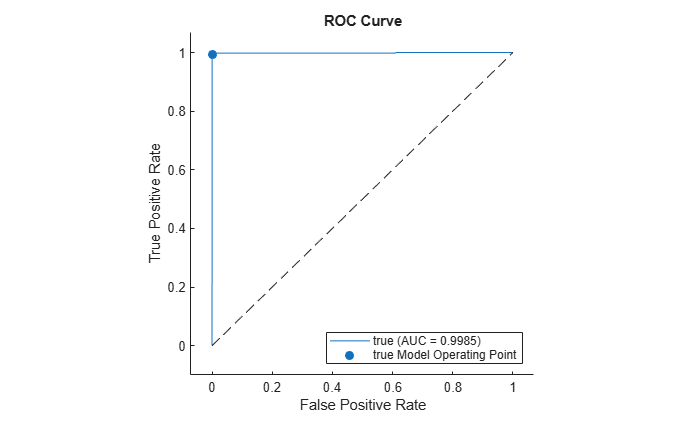The ROC curve indicates that the model classifies the test-sample observations almost perfectly.

To determine a good lasso-penalty strength for a linear classification model that uses a logistic regression learner, compare test-sample values of the AUC.

Load the NLP data set. Preprocess the data as in Predict Test-Sample Labels.

```load nlpdata Ystats = Y == 'stats'; X = X';```

Create a data partition that specifies to holdout 10% of the observations. Extract test-sample indices.

```rng(10); % For reproducibility Partition = cvpartition(Ystats,'Holdout',0.10); testIdx = test(Partition); XTest = X(:,testIdx); n = sum(testIdx)```
```n = 3157 ```
`YTest = Ystats(testIdx);`

There are 3157 observations in the test sample.

Create a set of 11 logarithmically-spaced regularization strengths from $1{0}^{-6}$ through $1{0}^{-0.5}$.

`Lambda = logspace(-6,-0.5,11);`

Train binary, linear classification models that use each of the regularization strengths. Optimize the objective function using SpaRSA. Lower the tolerance on the gradient of the objective function to `1e-8`.

```CVMdl = fitclinear(X,Ystats,'ObservationsIn','columns',... 'CVPartition',Partition,'Learner','logistic','Solver','sparsa',... 'Regularization','lasso','Lambda',Lambda,'GradientTolerance',1e-8)```
```CVMdl = ClassificationPartitionedLinear CrossValidatedModel: 'Linear' ResponseName: 'Y' NumObservations: 31572 KFold: 1 Partition: [1x1 cvpartition] ClassNames: [0 1] ScoreTransform: 'none' Properties, Methods ```

Extract the trained linear classification model.

`Mdl1 = CVMdl.Trained{1}`
```Mdl1 = ClassificationLinear ResponseName: 'Y' ClassNames: [0 1] ScoreTransform: 'logit' Beta: [34023x11 double] Bias: [-11.9079 -11.9079 -11.9079 -11.9079 -9.3362 -6.4290 -5.1424 -4.4991 -3.5732 -3.1742 -2.9839] Lambda: [1.0000e-06 3.5481e-06 1.2589e-05 4.4668e-05 1.5849e-04 5.6234e-04 0.0020 0.0071 0.0251 0.0891 0.3162] Learner: 'logistic' Properties, Methods ```

`Mdl` is a `ClassificationLinear` model object. Because `Lambda` is a sequence of regularization strengths, you can think of `Mdl` as 11 models, one for each regularization strength in `Lambda`.

Estimate the test-sample predicted labels and posterior class probabilities.

```[label,posterior] = predict(Mdl1,XTest,'ObservationsIn','columns'); Mdl1.ClassNames; posterior(3,1,5)```
```ans = 1.0000 ```

`label` is a 3157-by-11 matrix of predicted labels. Each column corresponds to the predicted labels of the model trained using the corresponding regularization strength. `posterior` is a 3157-by-2-by-11 matrix of posterior class probabilities. Columns correspond to classes and pages correspond to regularization strengths. For example, `posterior(3,1,5)` indicates that the posterior probability that the first class (label `0`) is assigned to observation 3 by the model that uses `Lambda(5)` as a regularization strength is 1.0000.

For each model, compute the AUC by using `rocmetrics`.

```auc = 1:numel(Lambda); % Preallocation for j = 1:numel(Lambda) rocObj = rocmetrics(YTest,posterior(:,:,j),Mdl1.ClassNames); auc(j) = rocObj.AUC(1); end```

Higher values of `Lambda` lead to predictor variable sparsity, which is a good quality of a classifier. For each regularization strength, train a linear classification model using the entire data set and the same options as when you trained the model. Determine the number of nonzero coefficients per model.

```Mdl = fitclinear(X,Ystats,'ObservationsIn','columns',... 'Learner','logistic','Solver','sparsa','Regularization','lasso',... 'Lambda',Lambda,'GradientTolerance',1e-8); numNZCoeff = sum(Mdl.Beta~=0);```

In the same figure, plot the test-sample error rates and frequency of nonzero coefficients for each regularization strength. Plot all variables on the log scale.

```figure yyaxis left plot(log10(Lambda),log10(auc),'o-') ylabel('log_{10} AUC') yyaxis right plot(log10(Lambda),log10(numNZCoeff + 1),'o-') ylabel('log_{10} nonzero-coefficient frequency') xlabel('log_{10} Lambda') title('Test-Sample Statistics') hold off```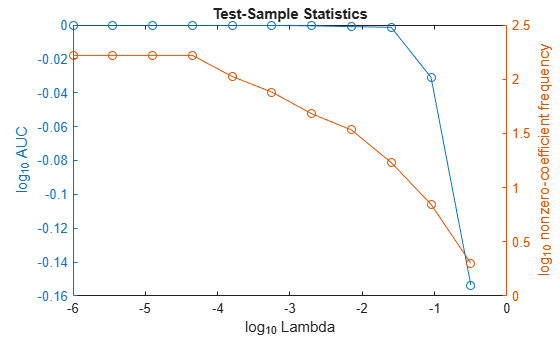Choose the index of the regularization strength that balances predictor variable sparsity and high AUC. In this case, a value between $1{0}^{-2}$ to $1{0}^{-1}$ should suffice.

`idxFinal = 9;`

Select the model from `Mdl` with the chosen regularization strength.

`MdlFinal = selectModels(Mdl,idxFinal);`

`MdlFinal` is a `ClassificationLinear` model containing one regularization strength. To estimate labels for new observations, pass `MdlFinal` and the new data to `predict`.

expand all

## Alternative Functionality

To integrate the prediction of a linear classification model into Simulink®, you can use the ClassificationLinear Predict block in the Statistics and Machine Learning Toolbox™ library or a MATLAB® Function block with the `predict` function. For examples, see Predict Class Labels Using ClassificationLinear Predict Block and Predict Class Labels Using MATLAB Function Block.

When deciding which approach to use, consider the following:

• If you use the Statistics and Machine Learning Toolbox library block, you can use the Fixed-Point Tool (Fixed-Point Designer) to convert a floating-point model to fixed point.

• Support for variable-size arrays must be enabled for a MATLAB Function block with the `predict` function.

• If you use a MATLAB Function block, you can use MATLAB functions for preprocessing or post-processing before or after predictions in the same MATLAB Function block.

## Version History

Introduced in R2016a